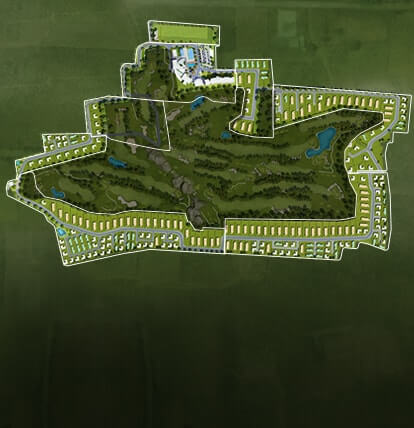NORTHZone 1
Zone 2
Zone 3
Zone 4
Zone 5
Zone 6
Zone 7
Zone 8ACRES

OPEN SPACE

• ## 10%

BUILT UP AREA

• ## 98

COURSE FACING PLOTS

• ## 144

PRIVATE PLOTS

• ## 11000 – 16000

SQFT PLOT RANGE

• ## 90,000

SQFT CLUBHOUSE

#### zone 12936.00SQ FT
PLOT RANGE
2936.00SQ FT
2936.00SQ FT
PLOT RANGE
2936.00SQ FT

#### zone 2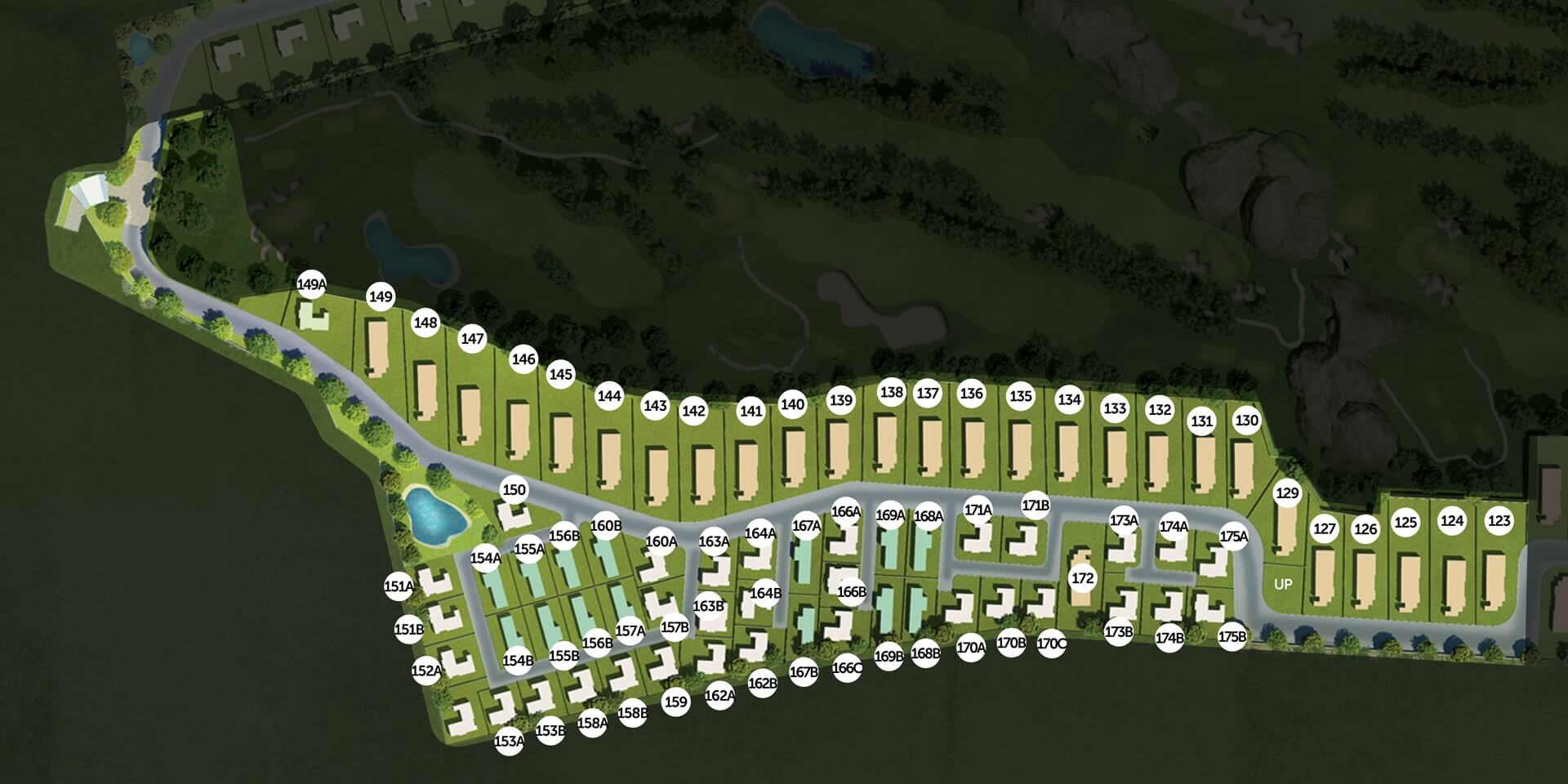2936.00SQ FT
PLOT RANGE
2936.00SQ FT
2936.00SQ FT
PLOT RANGE
2936.00SQ FT

#### zone 3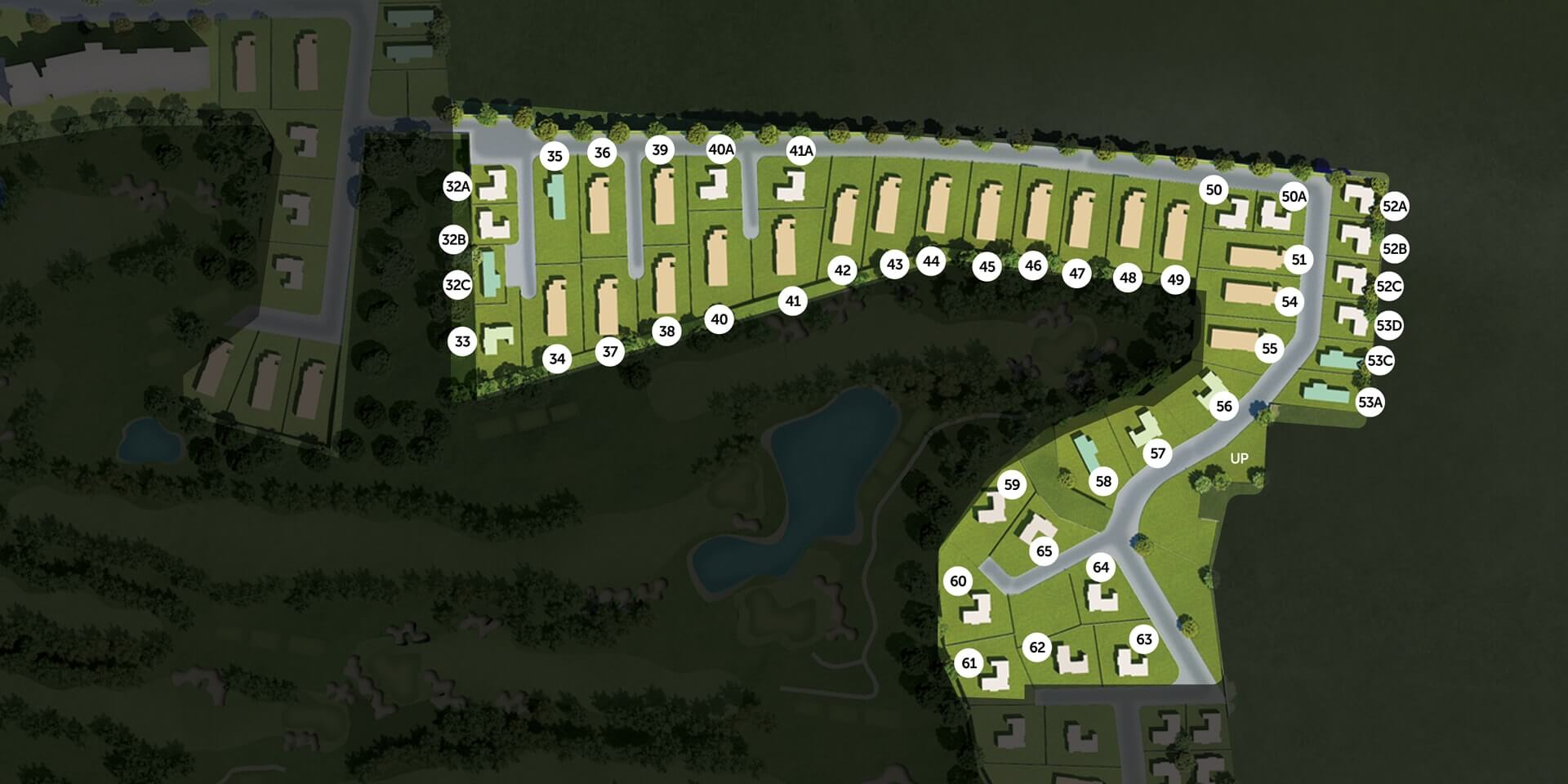2936.00SQ FT
PLOT RANGE
2936.00SQ FT
2936.00SQ FT
PLOT RANGE
2936.00SQ FT

#### zone 42936.00SQ FT
PLOT RANGE
2936.00SQ FT
2936.00SQ FT
PLOT RANGE
2936.00SQ FT

#### zone 52936.00SQ FT
PLOT RANGE
2936.00SQ FT
2936.00SQ FT
PLOT RANGE
2936.00SQ FT

#### zone 6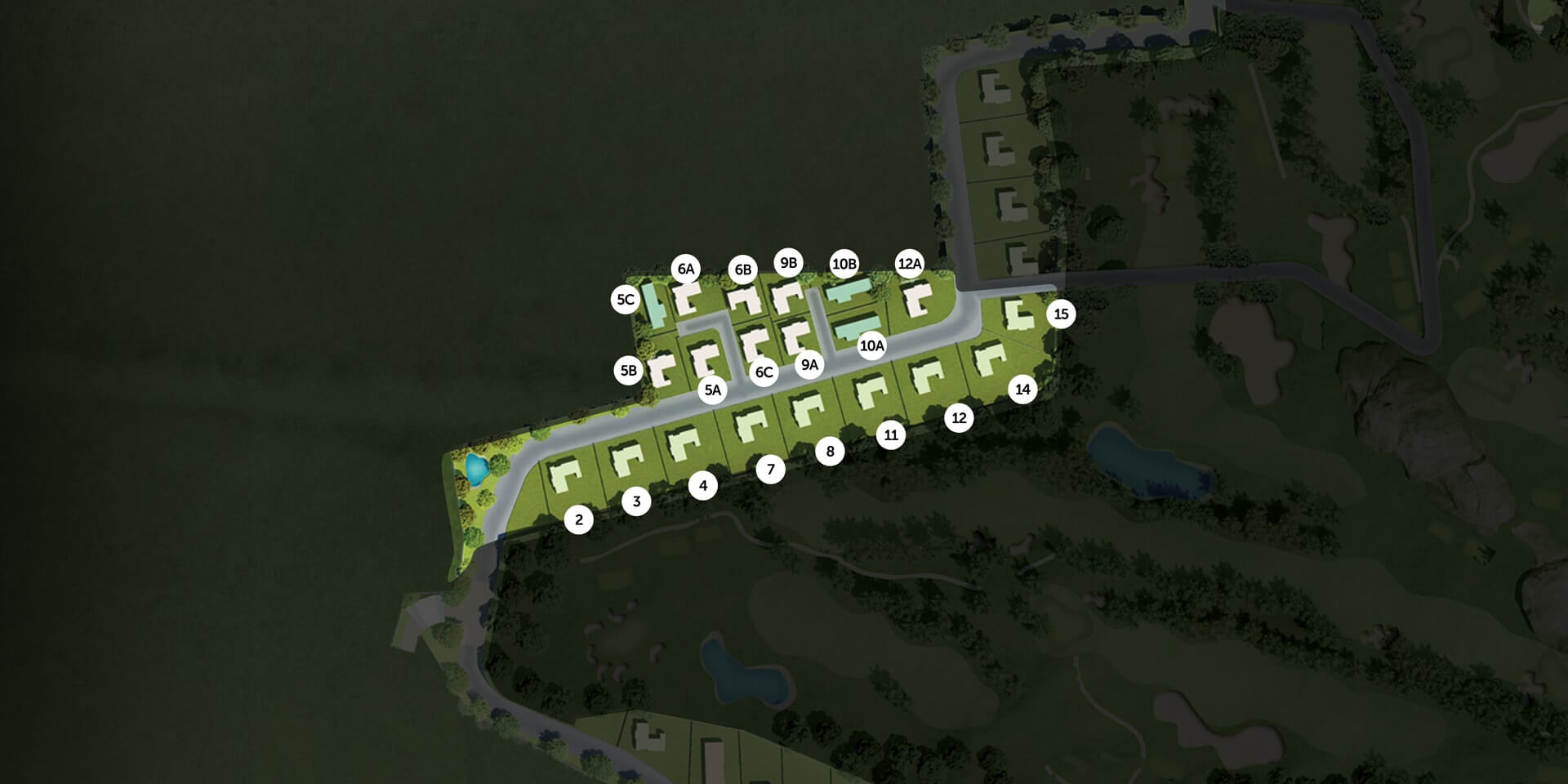2936.00SQ FT
PLOT RANGE
2936.00SQ FT
2936.00SQ FT
PLOT RANGE
2936.00SQ FT

#### zone 7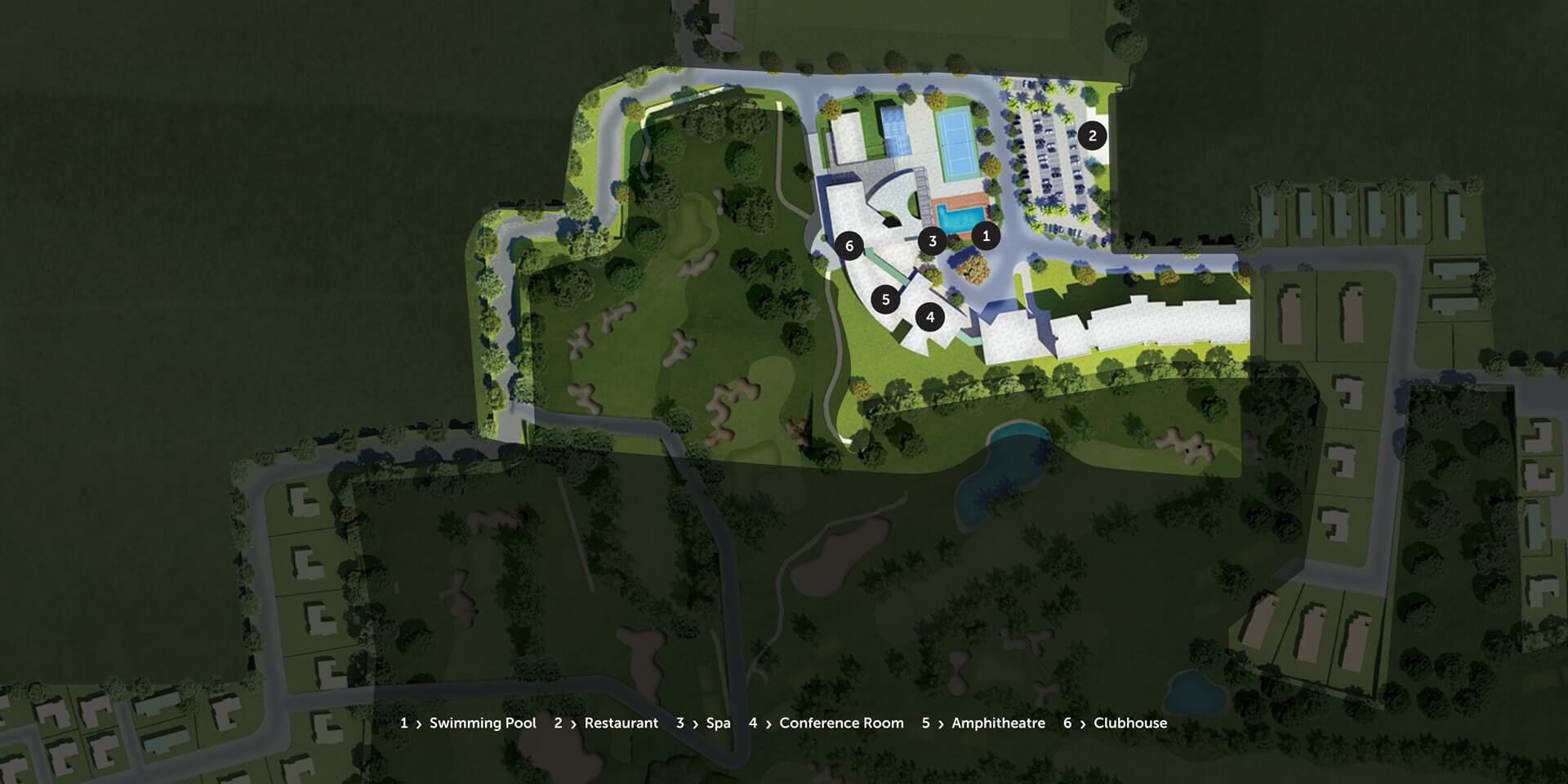2936.00SQ FT
PLOT RANGE
2936.00SQ FT
2936.00SQ FT
PLOT RANGE
2936.00SQ FT

#### zone 8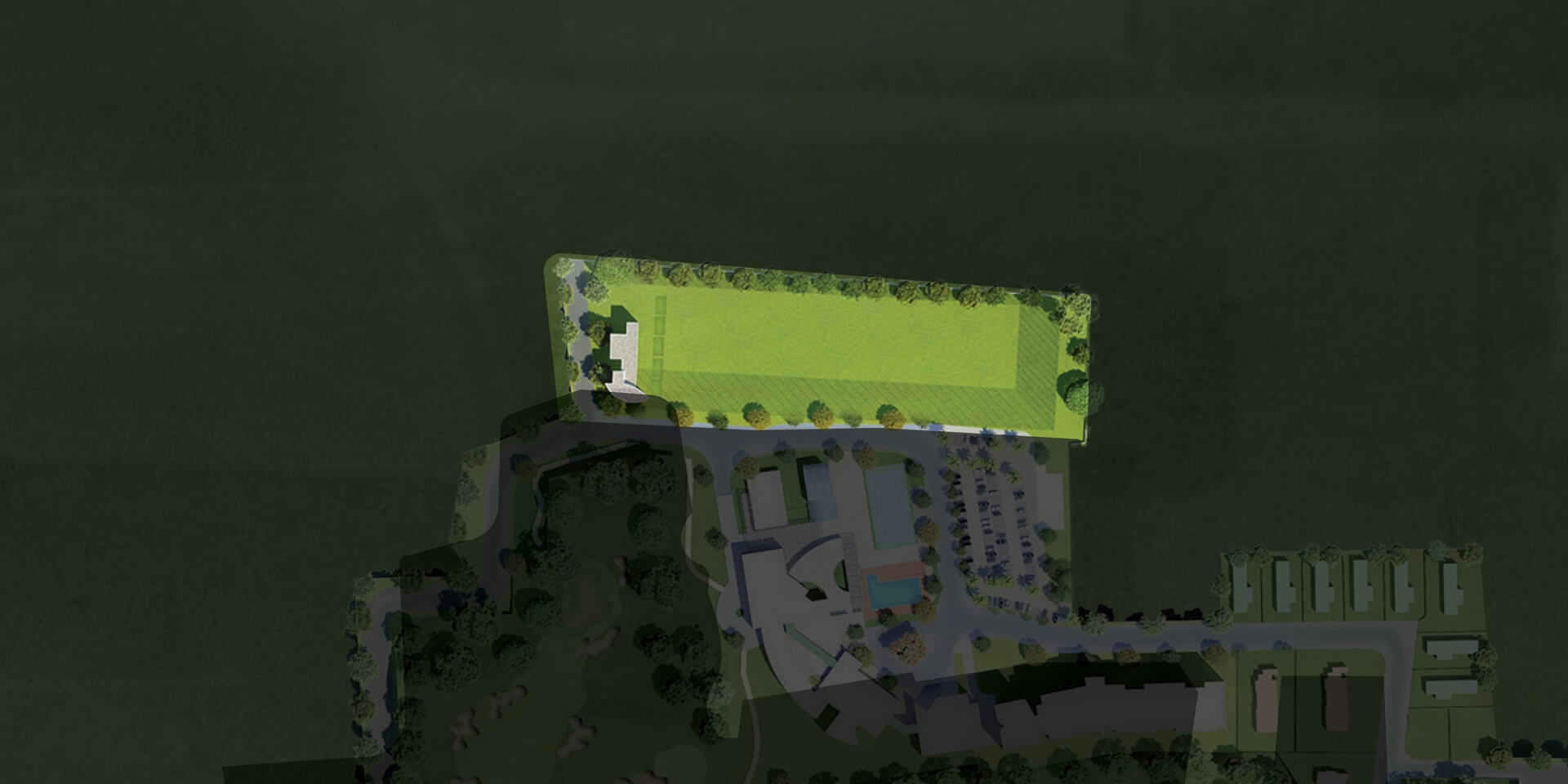2936.00SQ FT
PLOT RANGE
2936.00SQ FT
2936.00SQ FT
PLOT RANGE
2936.00SQ FT